# SSAT Upper Level Math : How to graph an ordered pair

## Example Questions

← Previous 1 3 4 5 6

### Example Question #5 : How To Graph An Ordered Pair

Which point best represents the coordinate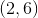?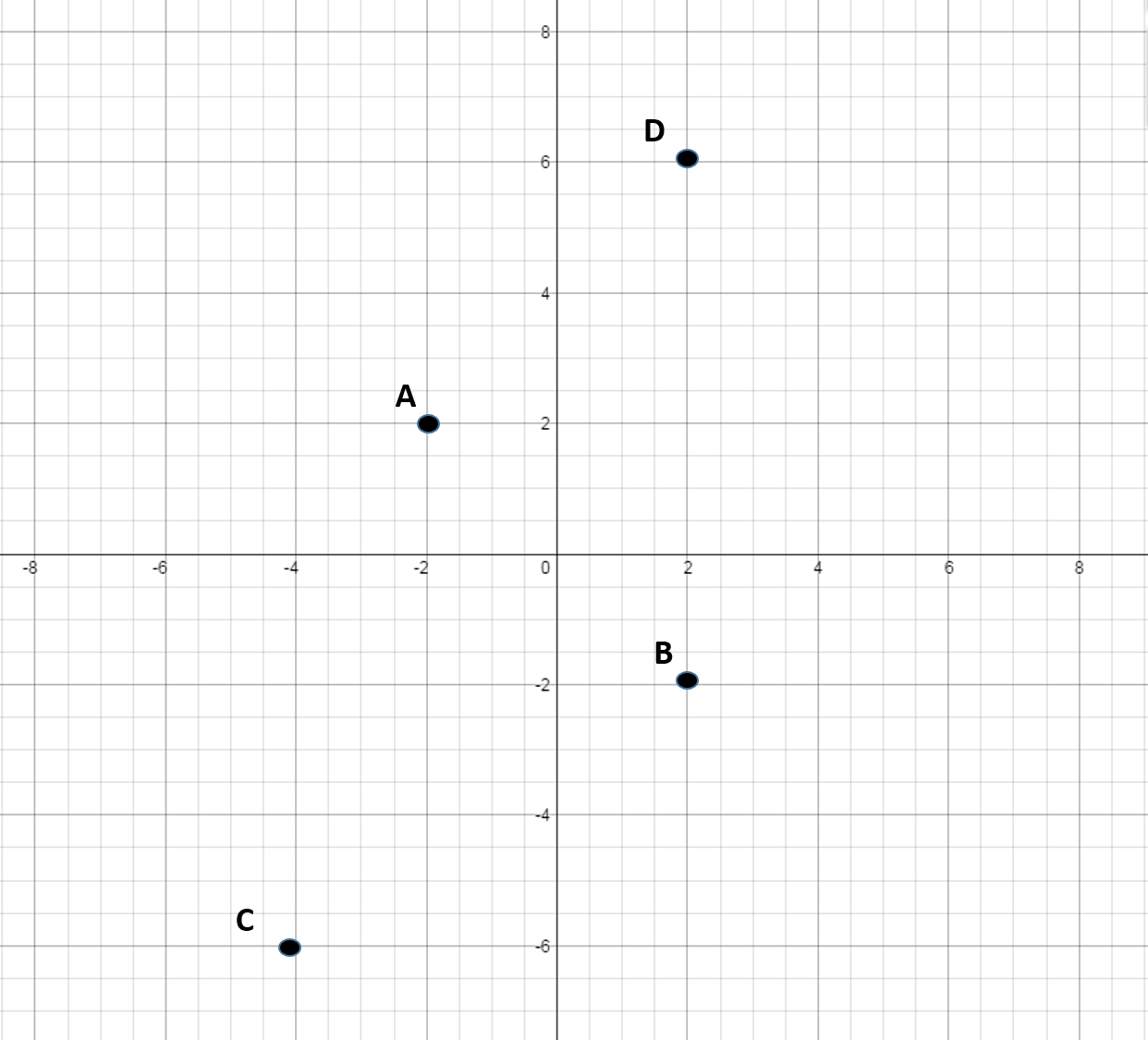B

D

A

C

D

Explanation:

To get to, move right along the x-axis by, then move up on the y-axis by.

The sign in front of each number represents which direction to go on the x or y-axis starting at the origin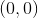. If the number is positive you go right on the x-axis or up on the y-axis. If the sign is negative then you move to the left on the x-axis or down on the y-axis.

### Example Question #6 : How To Graph An Ordered Pair

Which point best represents the coordinates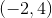?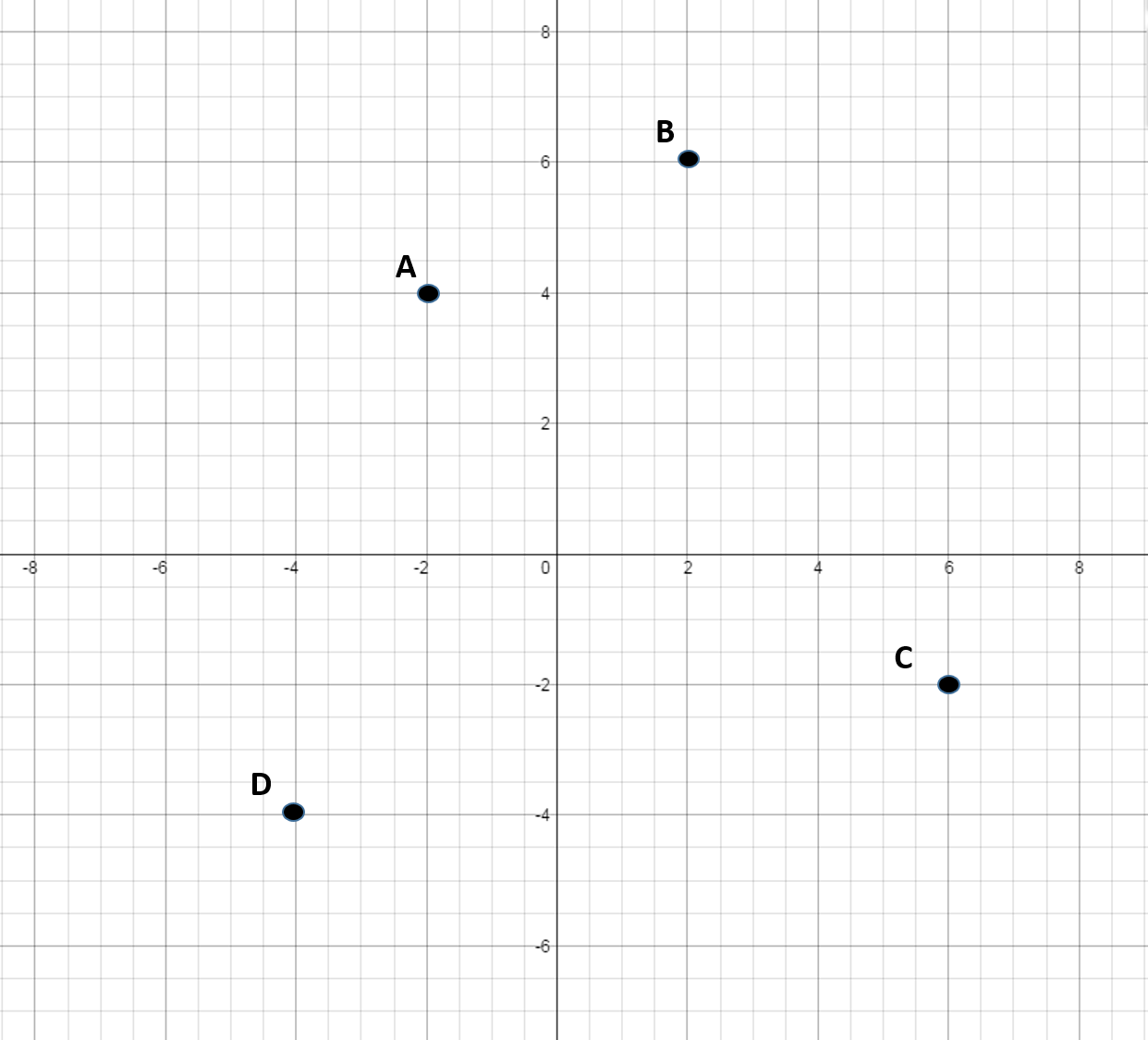A

B

C

D

A

Explanation:

The sign in front of each number represents which direction to go on the x or y-axis starting at the origin. If the number is positive you go right on the x-axis or up on the y-axis. If the sign is negative then you move to the left on the x-axis or down on the y-axis.

To get to, move left on the x-axis by, then move up on the y-axis by.

### Example Question #1 : How To Graph An Ordered Pair

Which point best represents the coordinate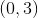?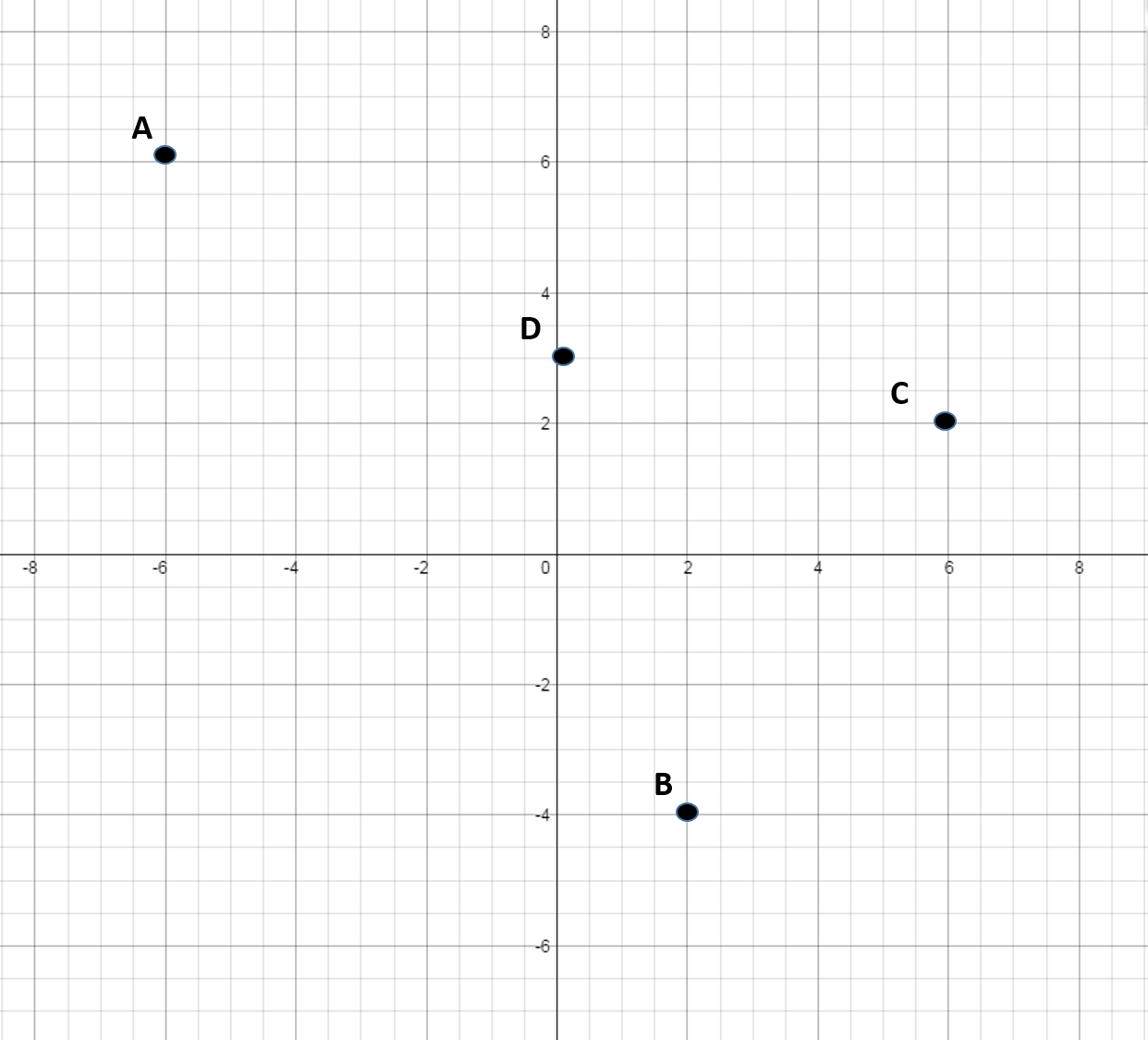B

D

C

A

D

Explanation:

The sign in front of each number represents which direction to go on the x or y-axis starting at the origin. If the number is positive you go right on the x-axis or up on the y-axis. If the sign is negative then you move to the left on the x-axis or down on the y-axis.

To get to, only move up byon the y-axis. Do not move along the x-axis because the x-coordinate is.

### Example Question #8 : How To Graph An Ordered Pair

Which point best represents the coordinate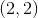?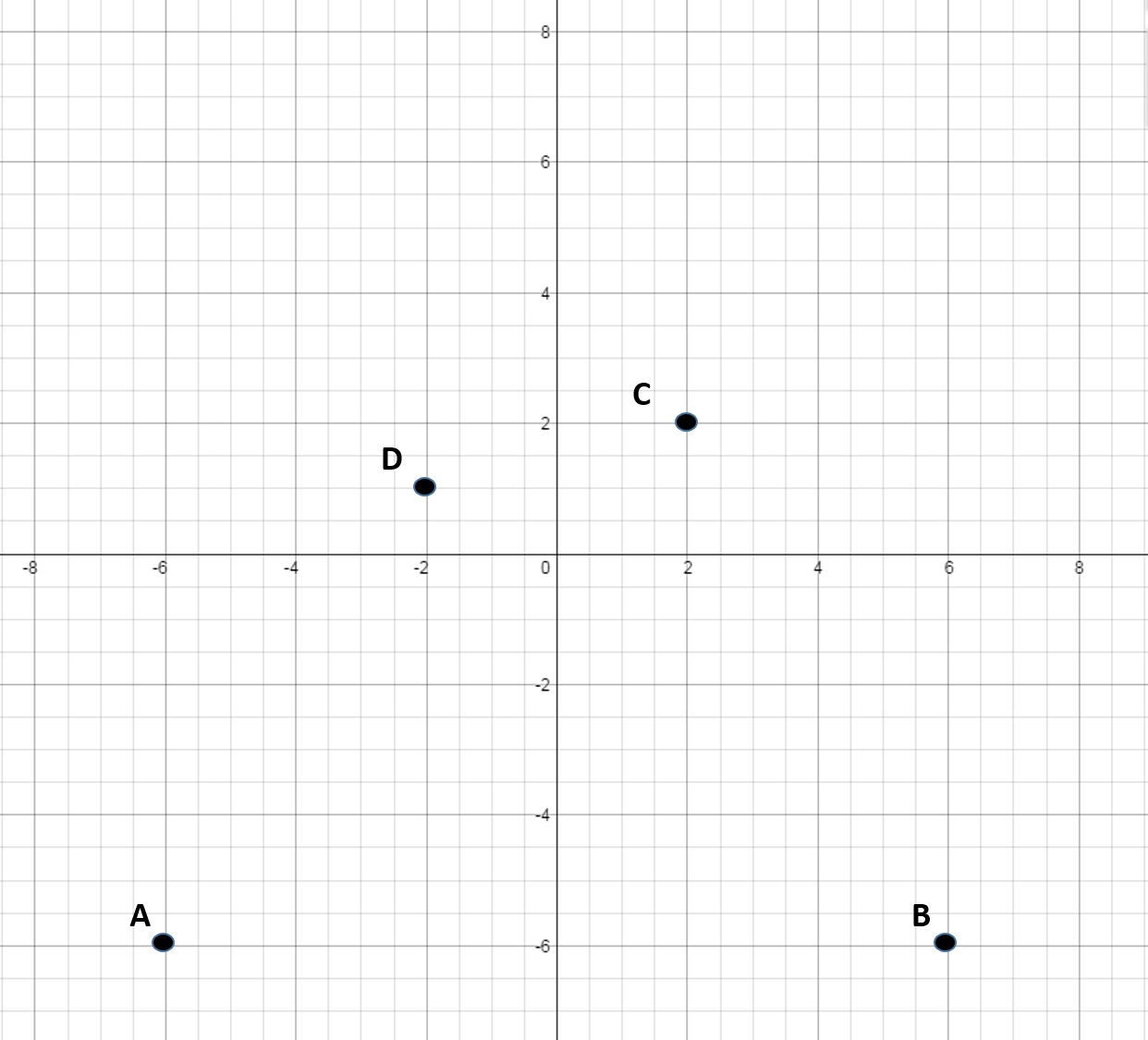D

B

C

A

C

Explanation:

To get to, move right on the x-axis bythen move up on the y-axis by.

The sign in front of each number represents which direction to go on the x or y-axis starting at the origin. If the number is positive you go right on the x-axis or up on the y-axis. If the sign is negative then you move to the left on the x-axis or down on the y-axis.

### Example Question #9 : How To Graph An Ordered Pair

Which point best represents the coordinate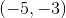?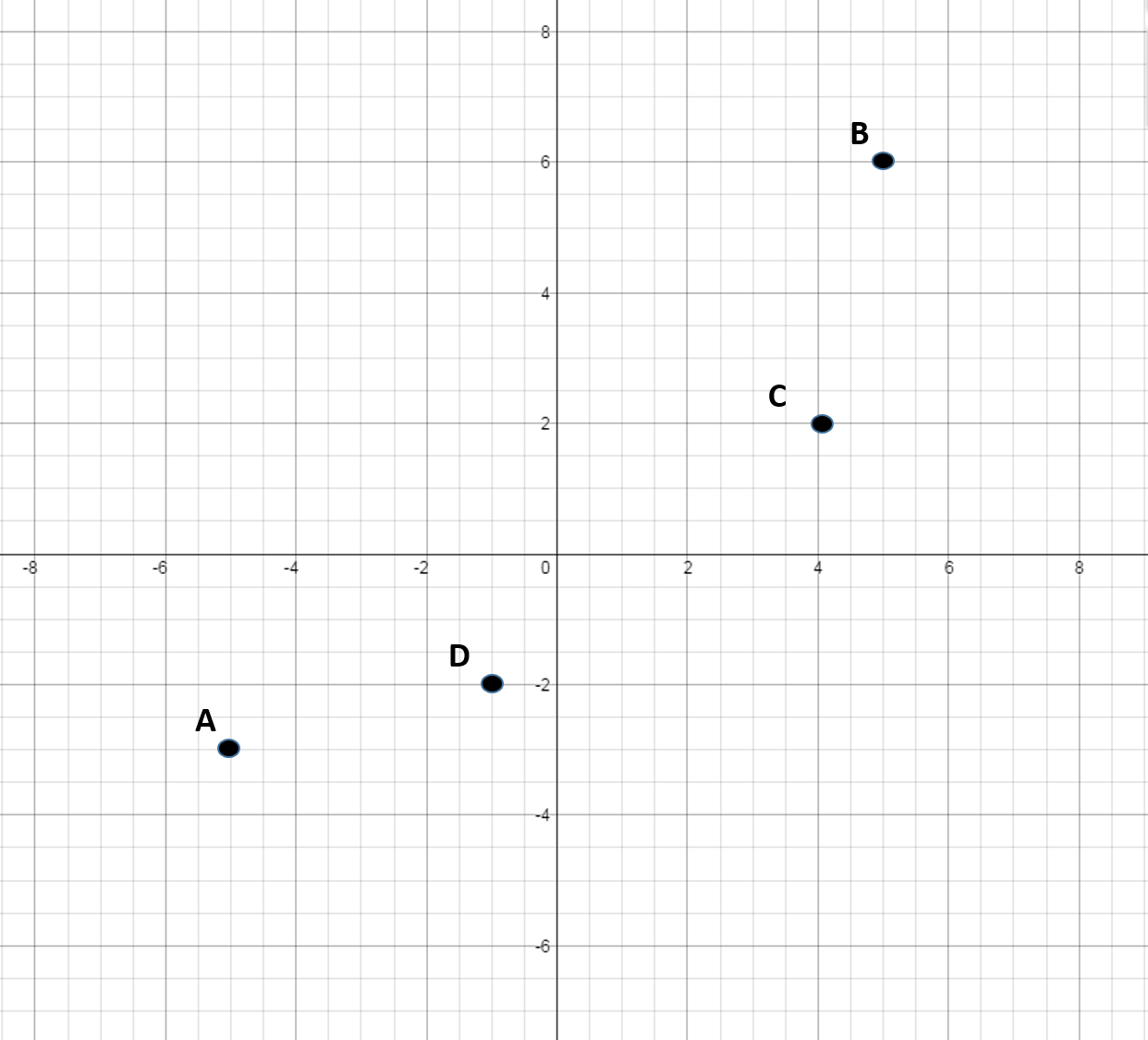B

D

A

C

A

Explanation:

To get to, move left on the x-axis bythen move down the y-axis by.

The sign in front of each number represents which direction to go on the x or y-axis starting at the origin. If the number is positive you go right on the x-axis or up on the y-axis. If the sign is negative then you move to the left on the x-axis or down on the y-axis.

### Example Question #10 : How To Graph An Ordered Pair

Which point best represents the coordinate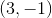?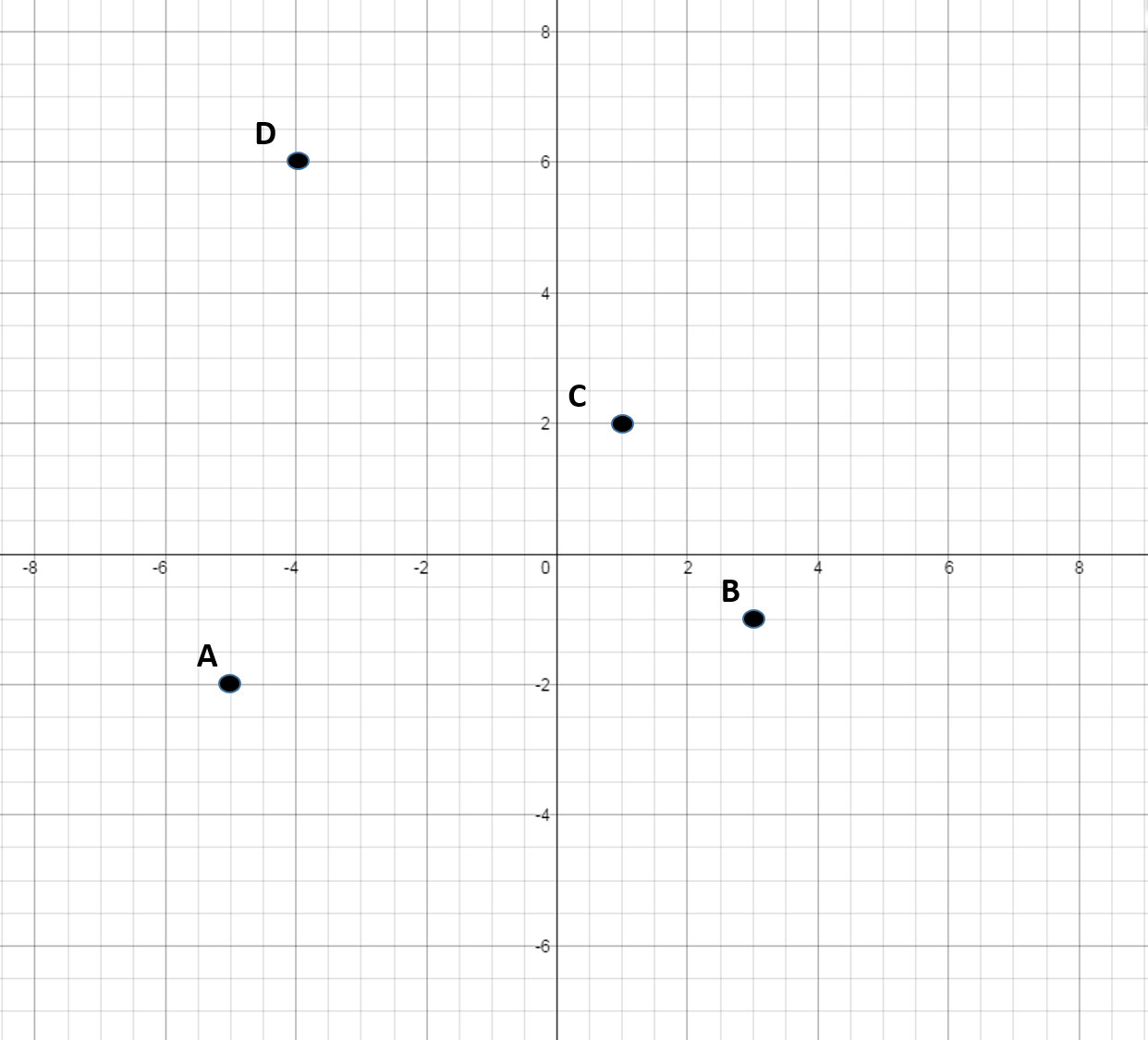A

D

C

B

B

Explanation:

The sign in front of each number represents which direction to go on the x or y-axis starting at the origin. If the number is positive you go right on the x-axis or up on the y-axis. If the sign is negative then you move to the left on the x-axis or down on the y-axis.

To get to, move right on the x-axis by, then move down on the y-axis by.

### Example Question #11 : How To Graph An Ordered Pair

Which point best represents the coordinate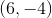?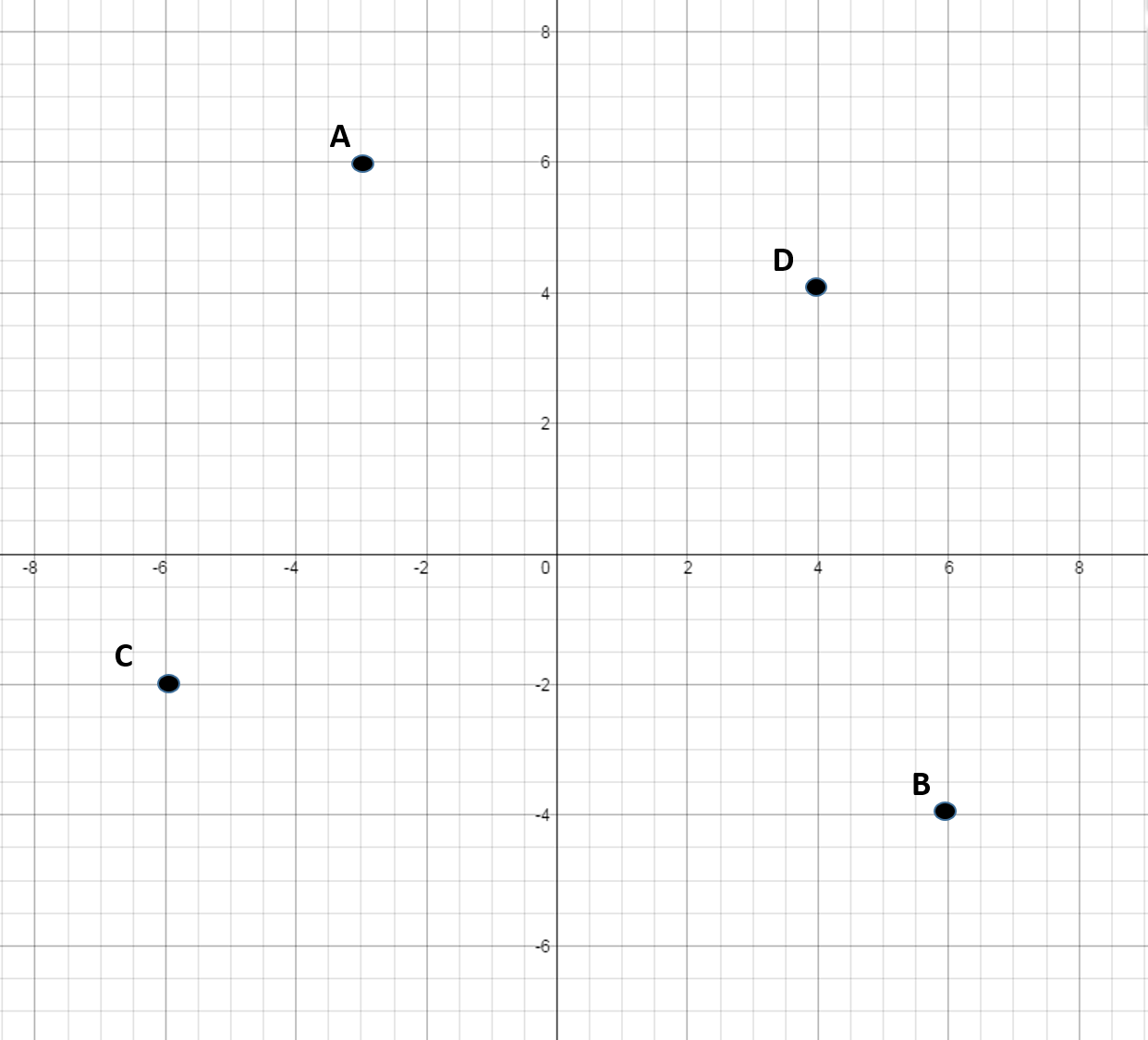C

B

D

A

B

Explanation:

The sign in front of each number represents which direction to go on the x or y-axis starting at the origin. If the number is positive you go right on the x-axis or up on the y-axis. If the sign is negative then you move to the left on the x-axis or down on the y-axis.

To get to, move right on the x-axis bythen move down on the y-axis by.

### Example Question #1 : Graphing

Which point best represents the coordinate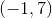?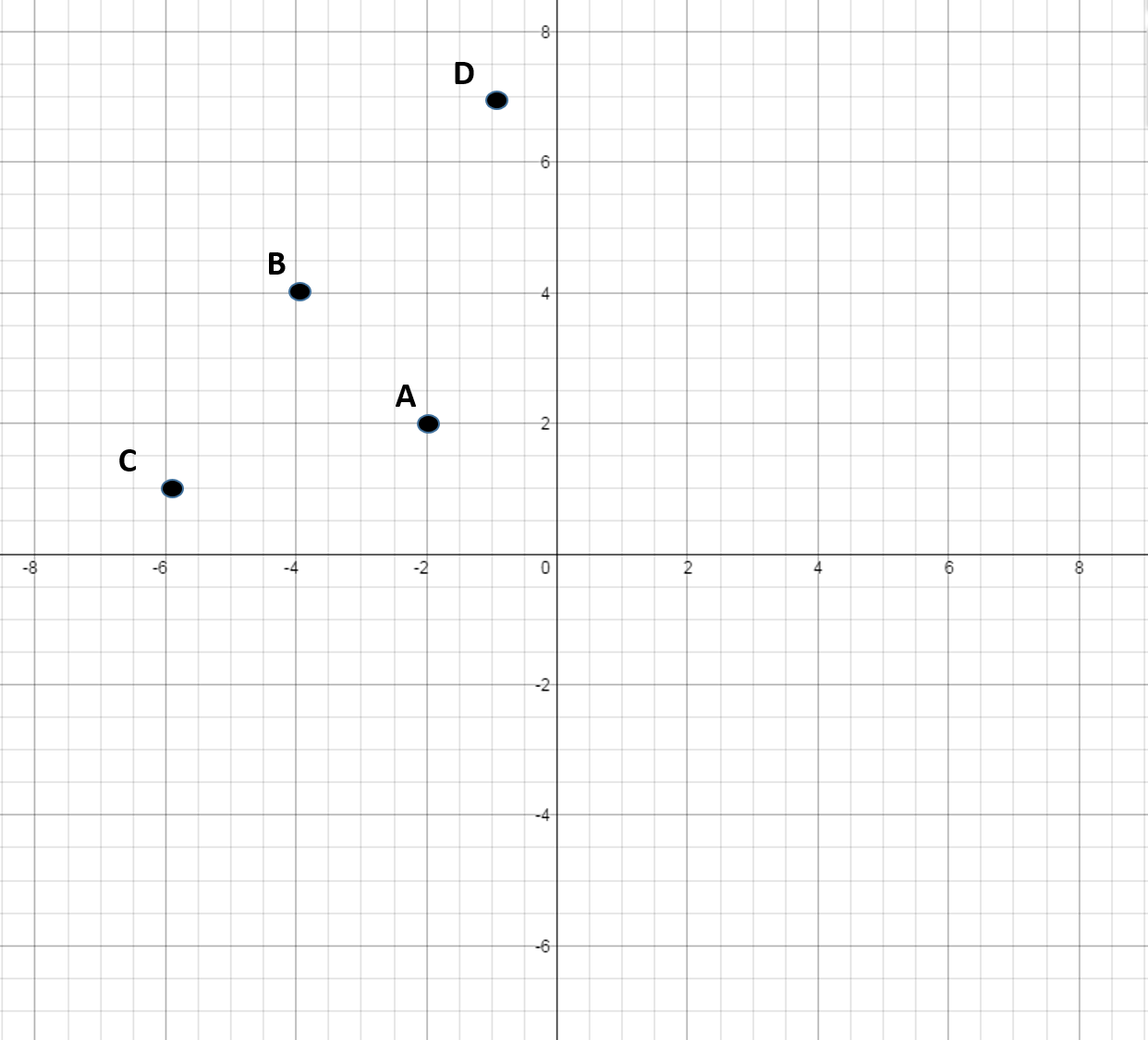B

A

C

D

D

Explanation:

The sign in front of each number represents which direction to go on the x or y-axis starting at the origin. If the number is positive you go right on the x-axis or up on the y-axis. If the sign is negative then you move to the left on the x-axis or down on the y-axis. The first term in the ordered pair is the movement in the x direction and the second point is the movement in the y direction.

To get to, move left on the x-axis by, then move up on the y-axis by.

### Example Question #13 : How To Graph An Ordered Pair

Which point best represents the coordinate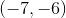?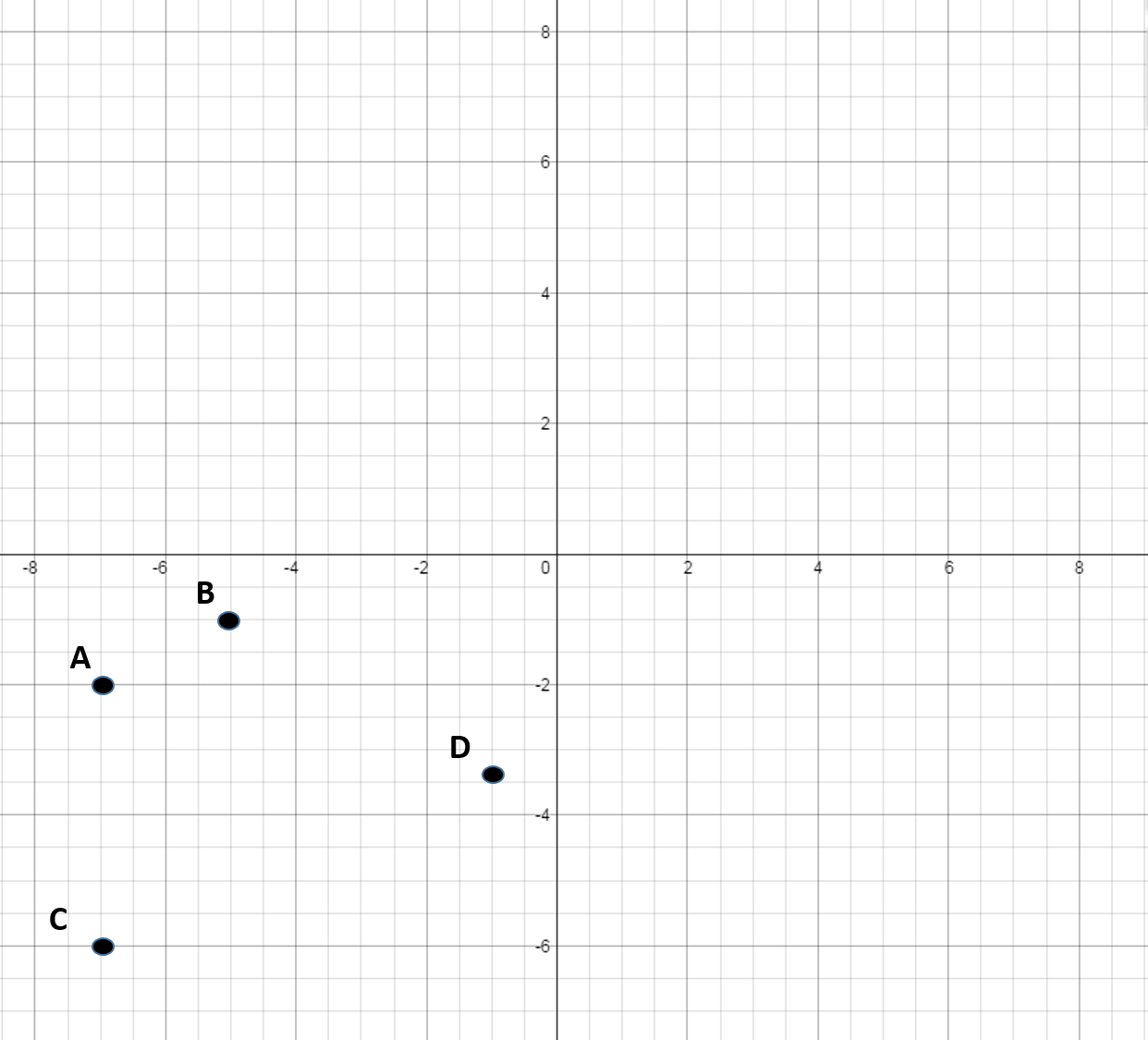D

A

C

B

C

Explanation:

To get to, move left on the x-axis by, then down on the y-axis by.

The sign in front of each number represents which direction to go on the x or y-axis starting at the origin. If the number is positive you go right on the x-axis or up on the y-axis. If the sign is negative then you move to the left on the x-axis or down on the y-axis. The first term in the ordered pair is the movement in the x direction and the second point is the movement in the y direction.

### Example Question #14 : How To Graph An Ordered Pair

Which point best represents the coordinate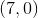?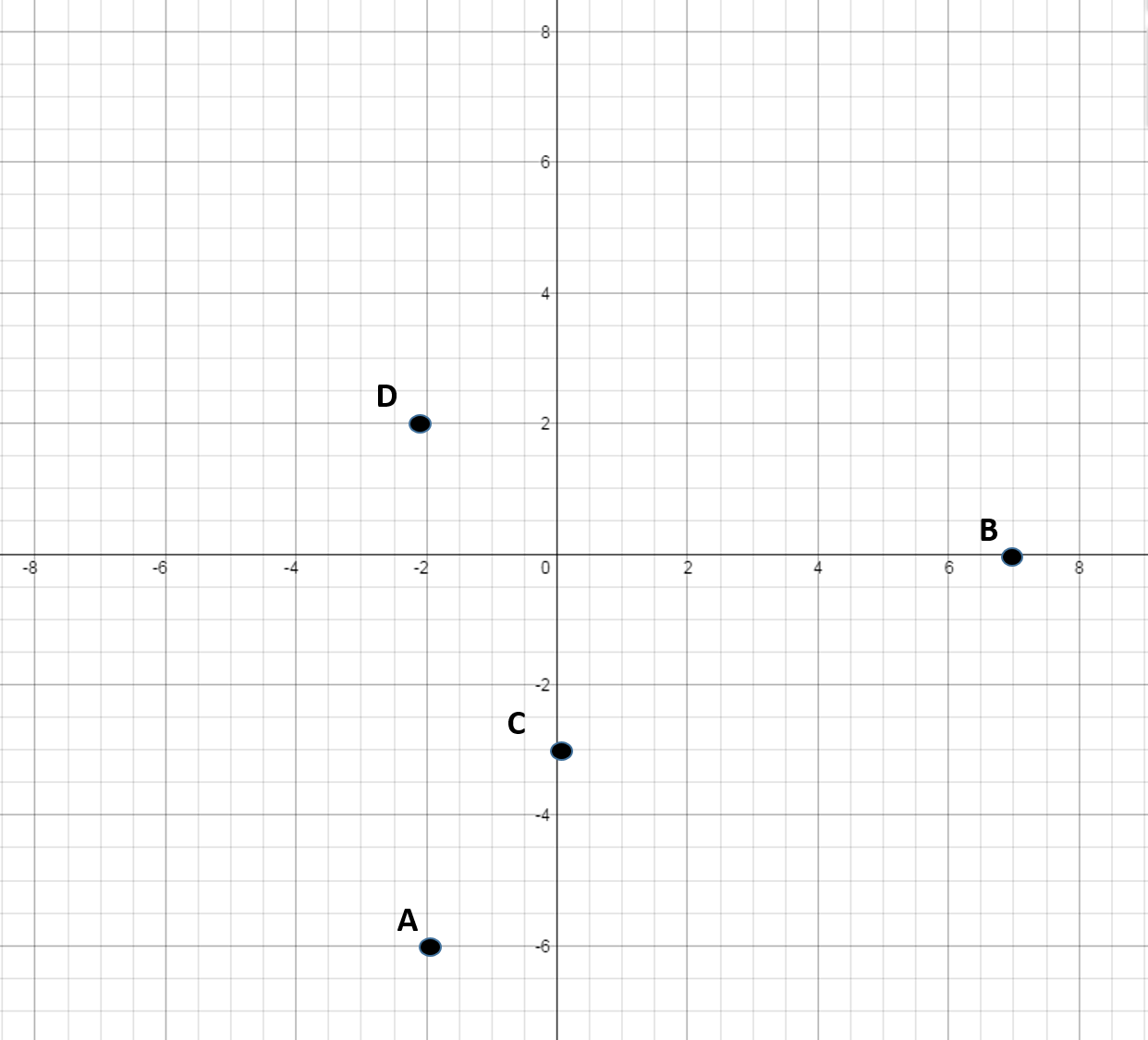D

B

C

A

The sign in front of each number represents which direction to go on the x or y-axis starting at the origin. If the number is positive you go right on the x-axis or up on the y-axis. If the sign is negative then you move to the left on the x-axis or down on the y-axis. The first term in the ordered pair is the movement in the x direction and the second point is the movement in the y direction.
To get to, only move right on the x-axis by.